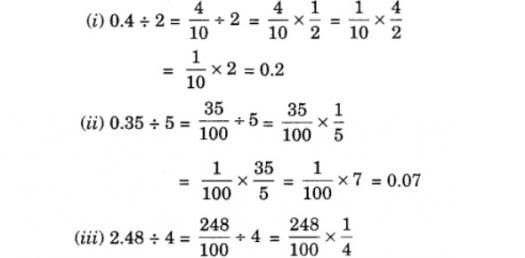# Do You Have IT In You? To Take This Class 7 Maths Quiz

10 Questions | Total Attempts: 476SettingsThis is a very good maths quiz and can train you for class 7 examinations. This quiz can also be taken by elders. This is very competitive and tough quiz!

• 1.
The digit 7 in the no. 27109 is replaced by 5.By how much will the no. decrease?????
• A.

2

• B.

2000

• C.

2109

• D.

5000

• 2.
Which of these nos. is less than -2020?
• A.

-3030

• B.

-2002

• C.

2020

• D.

0

• 3.
By how much is 50,000,000 greater than 5,00,000?
• A.

5 lakhs

• B.

45 lakhs

• C.

50 lakhs

• D.

4 crore

• 4.
31 is a factor of 1767 and 3543 is a multiple of 1767.which of these is true?
• A.

31 is a factor of 3534

• B.

31 is a multiple of 3534

• C.

1767 is a common factor of 31 & 3534

• D.

31 is a common multiple of 1767 & 3534

• 5.
Which of the following fractiojns is nearest to 2?
• A.

1/2 + 1/4

• B.

1/4 +1/6

• C.

2/3+6/7

• D.

2/5+1/10

• 6.
Which of these is the same as 23 1/19?
• A.

23+1/19

• B.

23-1/19

• C.

23*1/19

• D.

23/1/19

• 7.
What can be said about the number obtained on sub (-2010 from 2010)??
• A.

It is 0

• B.

It is smaller than -2010

• C.

It is greater than 2010

• D.

It is a positive number smaller than 2010

• 8.
Antra cal. the product 2222*2223*22*24 and got a number.What will be the remainder when the product is divided by 10?
• A.

2

• B.

3

• C.

4

• D.

8

• 9.
Saba has 10 stamps more than Amrita.Daya has 10 stamps less than Amrita . Which of the following is true??
• A.

Saba has 20 stams more than Daya

• B.

Saba has 10 stamps more than Daya

• C.

Saba=Daya

• D.

Cant say

• 10.
If the population of Iceland is roughly 319,000, which of these is the approx number of internet users in Iceland
• A.

319

• B.

403

• C.

319*403

• D.

319,000*403

Related TopicsBack to top